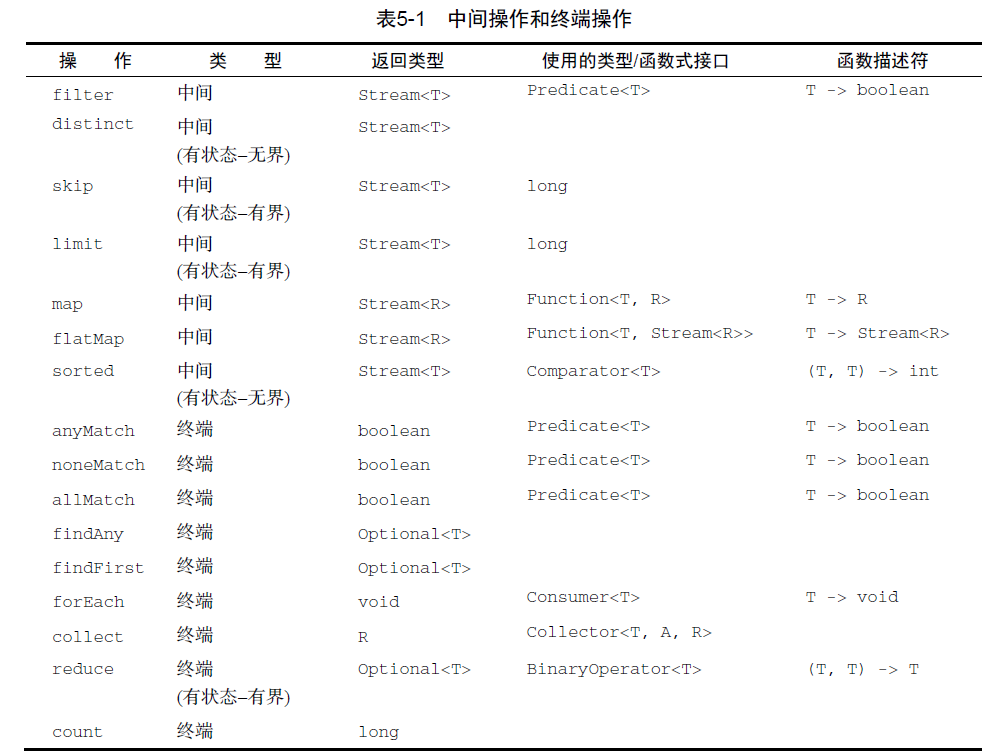# Java8新特性之Stream

2019/04/10 10:10

Java8新特性之Lambda

## 哪里用Stream

### 集合迭代

• 优点
• 对于程序的掌控更高
• 性能强（如果算法功力深厚）
• 缺点
• 很多重复的模板代码
• 需要很多中间临时变量来减少遍历次数
• 性能完全取决于程序员水平，烧脑
• 代码不易读

• 优点
• 代码好读
• 简单，只需要提供处理逻辑
• 缺点
• 有些情况性能比外部迭代差一点点
• 在使用foreach时不能对元素进行赋值操作

## 为什么要Stream

• 流水线的方式处理集合，结合Lambda爽歪歪
• 代码超短超好读
• Stream的开始到结束就相当于一次遍历，允许我们在遍历中拼接多个操作
• 强调的是对集合的计算逻辑，逻辑可以多次复用
• 特别繁重的任务可以很轻松的转为并行流，适合类似于大数据处理等业务
• 成本非常小的实现并行执行效果

## 怎么用Stream

• 开始操作：读取数据源（如集合）
• 中间操作：组装中间操作链，形成一条流的流水线
• 终端操作：一次迭代执行流水线，结束流，并生成结果

### 中间操作和终端操作

• 中间操作：流水线的部件，返回的是this，也就是Stream，此时迭代并没有执行
• 终端操作：流水线真正开始执行，返回的是处理结果，终端操作过后流关闭
• 无状态操作：例如对集合中所有元素做转换，或者过滤，不用保存别的元素的处理结果
• 有状态操作：例如排序，去重操作，需要保存之前集合中元素的状态
• 非短路操作：按部就班完成迭代，返回处理结果
• 短路操作：只有一达到预设的条件，立刻停止并返回### 并行流

• 并行流是通过 fork/join 线程池来实现的，该池是所有并行流共享的。默认情况，fork/join 池会为每个处理器分配一个线程。假设你有一台16核的机器，这样你就只能创建16个线程。而与此同时其他任务将无法获得线程被阻塞住，所以使用并行流要结合机器和业务场景。
• 避免在并行流中改变共享状态，小心使用有状态的操作
• 并行流并不一定就快，要将多个线程的执行结果汇总

## 实战

### 开始操作

#### 生成空流

``````Stream<Object> empty = Stream.empty();
``````

#### 值生成流

``````Stream<String> stringStream = Stream.of("1", "2", "3");
``````

#### 数组生成流

``````String[] strings = { "1", "2", "3"};
Stream<String> stream = Arrays.stream(strings);
``````

#### 集合生成流

``````List<String> strings1 = Arrays.asList("1", "2", "3");
Stream<String> stream1 = strings1.stream();
``````

#### 文件生成流

``````Stream<String> lines = Files.lines(Paths.get("/c/mnt/"));
``````

#### 函数生成流（无限流）

``````// 无限流，流从0开始，下面的每个元素依次加2
Stream<Integer> iterate = Stream.iterate(0, num -> num + 2);
// 无限流，流中每个元素都是 0~1 随机数
Stream<Double> generate = Stream.generate(Math::random);
``````

#### 数值范围生成流

``````// 生成0到10的int流
IntStream intStream = IntStream.rangeClosed(0, 10);
// 生成0到9的int流
IntStream intStream1 = IntStream.range(0, 10);
``````

#### 手动生成流

``````// 生成有字符串a和数字1的异构的流
``````

#### 合并两个流

``````Stream.concat(
Stream.of("1", 22, "333"),
Stream.of("1", 22, 333)
).forEach(System.out::print);
``````

### 中间操作

#### 过滤filter

``````// 过滤所有空字符串，注意是返回 true的留下
Stream.of("1", null, "2", "", "3")
.filter(StringUtils::isNotEmpty)
.forEach(System.out::print);
``````

#### 去重distinct

``````// 去掉重复的2
Stream.of("1", "2", "2", "2", "3")
.distinct()
.forEach(System.out::print);
``````

#### 跳过skip

``````// 跳过前两个元素
Stream.of("1", "2", "3", "4", "5")
.skip(2)
.forEach(System.out::print);
``````

#### 截短limit

``````// 与skip相反，只留下前2个
Stream.of("1", "2", "3", "4", "5")
.limit(2)
.forEach(System.out::print);
``````

#### 映射map

``````@Data
@AllArgsConstructor
public class Person {
private String name;
}

// 将流中每个字符串转为Person实例
Stream.of("1", "2", "3")
.map(Person::new)
.forEach(System.out::print);

// 将流每个字符串变成其长度，为了避免自动拆箱，使用mapToInt转为IntStream
Stream.of("1", "2", "3")
.mapToInt(String::length)
.forEach(System.out::print);
``````

#### 扁平映射flatMap

``````// 每个字符串按照逗号分隔合并成一个流
Stream.of("1,2,3", "4,5,6", "7,8,9")
.flatMap(a -> Stream.of(a.split(",")))
.forEach(System.out::print);
``````

#### 排序sorted

``````Stream.of("4", "3", "5")
.sorted()
.forEach(System.out::print);

Stream.of("4", "3", "5")
.sorted(Comparator.naturalOrder())
.forEach(System.out::print);
``````

#### 并行流相关parallel，sequential，unordered

``````// 串行流转并行流
Stream.of("1", "2", "3").parallel();
// 并行流转串行流
Arrays.asList("1", "2", "3").parallelStream().sequential();

// 在并行流中加上unordered，使得流变成无序，提供并行效率，此时limit相当于随机取2个元素
Arrays.asList("1", "2", "3", "4", "5")
.parallelStream()
.unordered()
.limit(2).forEach(System.out::print);
``````

#### 调试peek

``````// 每个元素会打印两遍
Stream.of("1", "2", "3").peek(System.out::print).forEach(System.out::print);
``````

### 终端操作

#### 遍历foreach

``````Stream.of("1", "2", "3").forEach(System.out::print);

// 并行流中使用用于保持有序
Arrays.asList("1", "2", "3").parallelStream().forEachOrdered(System.out::print);
``````

#### 通用统计count，max，min

``````// 总个数，返回true|false
Stream.of("1", "2", "3").count();
// 最大元素，返回Optional
Stream.of("1", "2", "3").max(Comparator.naturalOrder());
// 最小元素，返回Optional
Stream.of("1", "2", "3").min(Comparator.naturalOrder());
``````

#### 数值流特有统计sum，average，summaryStatistics

``````// 累加求和
Stream.of("1", "2", "3").mapToInt(Integer::valueOf).sum();
// 求平均数
Stream.of("1", "2", "3").mapToInt(Integer::valueOf).average();
// 总和，最大值，最小值，平均数，总个数，应有尽有
Stream.of("1", "2", "3").mapToInt(Integer::valueOf).summaryStatistics();
``````

#### 匹配match

``````// 是否有长度大于 2 的字符串
Stream.of("1", "22", "333").anyMatch(s -> s.length() > 2);
// 是否一个长度大于 2 的字符串也没有
Stream.of("1", "22", "333").noneMatch(s -> s.length() > 2);
// 是否字符串长度全大于 2
Stream.of("1", "22", "333").allMatch(s -> s.length() > 2);
``````

#### 查找find

``````// 找到流中任意一个元素，普通流一般也返回第一个元素，并行流中返回任意元素
Stream.of("1", "22", "333").findAny();
// 找到流中第一个元素，普通流和并行流都一样
Stream.of("1", "22", "333").findFirst();
``````

#### 汇总collect

``````// 将结果汇总成一个list
Stream.of("1", "22", "333").collect(Collectors.toList());
``````

#### 归约reduce

``````// 0作为起始的pre，流的结果等于pre乘以自身再加一，直到curr到达最后一个元素
// (1) pre = 0 curr = 1 计算 pre = 0 * 1 + 1 = 1
// (2) pre = 1 curr = 2 计算 pre = 1 * 2 + 1 = 3
// (3) pre = 3 curr = 3 计算 pre = 3 * 3 + 1 = 10
// (4) pre = 10 curr = null 返回结果 10
IntStream.of(1, 2, 3).reduce(0, (pre, curr) -> pre * curr + 1);

// 当然如果不设置初始值，流中第一个元素就是pre
IntStream.of(1, 2, 3).reduce((pre, curr) -> pre * curr + 1);
``````

#### 转数组toArray

``````Stream.of("1", "22", "333").toArray();
``````

#### 获得迭代器iterator

``````Stream.of("1", "2", "3").iterator();
``````

#### 获得并行可分迭代器spliterator

``````Stream.of("1", "2", "3").spliterator();
``````

#### 流类型判断isParallel

``````Stream.of("1", "2", "3").isParallel();
``````

### 收集器进阶

#### 转常用集合

``````// 转list
List<String> collect = Stream.of("1", "2", "3").collect(Collectors.toList());

// 转set
Set<String> collect = Stream.of("1", "2", "3").collect(Collectors.toSet());

// 转map，key为字符串长度，value为字符串本身
Map<Integer, String> collect = Stream.of("1", "2", "3")
.collect(Collectors.toMap(String::length, Function.identity()));

// 转并发版map，key为字符串长度，value为字符串本身
Map<Integer, String> collect = Stream.of("1", "2", "3")
.collect(Collectors.toConcurrentMap(String::length, Function.identity()));

// 转指定类型集合
ArrayList<String> collect = Stream.of("1", "2", "3")
.collect(Collectors.toCollection(ArrayList::new));
``````

#### 拼接字符串

``````// 拼接成一个字符串
String collect = Stream.of("1", "2", "3").collect(Collectors.joining());

// 拼接成一个字符串，逗号分隔
String collect = Stream.of("1", "2", "3").collect(Collectors.joining(","));
``````

#### 统计

``````// 和终端操作中的 max 等价
Stream.of("1", "2", "3").collect(Collectors.maxBy(Comparator.naturalOrder()));

// 和终端操作中的 min 等价
Stream.of("1", "2", "3").collect(Collectors.minBy(Comparator.naturalOrder()));

// 和终端操作中的 count 等价
Stream.of("1", "2", "3").collect(Collectors.counting(Integer::valueOf));

// 和数值流终端操作中的 sum 等价
Stream.of("1", "2", "3").collect(Collectors.summingInt(Integer::valueOf));

// 和数值流终端操作中的 average 等价
Stream.of("1", "2", "3").collect(Collectors.averagingInt(Integer::valueOf));

// 和数值流终端操作中的 summaryStatistics 等价
Stream.of("1", "2", "3").collect(Collectors.summarizingInt(Integer::valueOf));
``````

#### 分组

``````// 以字符串长度分成3组，map的key为长度，value为对应长度字符串list
Map<Integer, List<String>> collect = Stream.of("1", "22", "33", "4", "555")
.collect(Collectors.groupingBy(String::length));

// 以字符串长度分成3组，map的key为长度，value为对应的元素个数
Map<Integer, Long> collect = Stream.of("1", "22", "33", "4", "555")
.collect(Collectors.groupingBy(String::length, Collectors.counting()));

// 这个例子没有实际意义，展示我们可以进行二级分组，长度分组完毕，再组内以hash值分组
Map<Integer, Map<Integer, List<String>>> collect = Stream.of("1", "22", "33", "4", "555")
.collect(Collectors.groupingBy(
String::length,
Collectors.groupingBy(String::hashCode)
));
``````

#### 分区

``````// 以长度大于2为标准分区
Map<Boolean, List<String>> collect = Stream.of("1", "22", "33", "4", "555")
.collect(Collectors.partitioningBy(s -> s.length() > 2));
``````

#### 归约

``````// 和终端操作 reduce 等价
Stream.of("1", "22", "33", "4", "555")
.collect(Collectors.reducing(0, Integer::valueOf, Integer::sum))
``````

#### 多操作连接

``````// 把流汇总成list，然后再求出其容量
Integer collect = Stream.of("1", "22", "33", "4", "555")
.collect(Collectors.collectingAndThen(Collectors.toList(), List::size));
``````

#### 自定义

• supplier：提供一个容器 A 装结果
• accumulator：累加器，将元素累加进刚才创建的容器
• combiner：合并容器的结果
• finisher：完成操作，将 A 转为 R 返回
• characteristics：是个定义标识的方法
• UNORDERED：结果不受流中项目的遍历和累积顺序的影响
• CONCURRENT：accumulator函数可以从多个线程同时调用
• IDENTITY_FINISH：表示 finisher 没做任何事情，直接返回了累加的结果，也就是A和R相同
``````public static final Collector<String, List<String>, List<String>> myToList = Collector.of(
// supplier： 创建 A（ArrayList）
ArrayList::new,
// accumulator：把每个元素放入 A 中
// 如果不想支持并行可以写个空，或抛UnsupportedOperationException异常
(listA, listB) -> {
return listA;
},
// finisher：不做任何事情，直接返回 A
Function.identity(),
// characteristics...：表示 A R 类型相同， 且支持并行流
Collector.Characteristics.IDENTITY_FINISH,
Collector.Characteristics.CONCURRENT
);

// 自定义收集器转成list
List<String> collect = Stream.of("1", "22", "33", "4", "555").collect(myToList);
``````

0
0 收藏

### 作者的其它热门文章0 评论
0 收藏
0